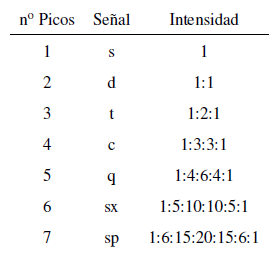From the above discussion it can be deduced that a proton produces a signal with a number of peaks one unit greater than the number of neighboring hydrogens. In the following image we can see the peaks produced by a hydrogen $H^b$ when coupled with a number of variable hydrogens $H^a$The intensity of the peaks of a signal are given by Pascal's triangle (Tartaglia)Two considerations must be taken into account when applying the N+1 rule:

• In molecules of the type $A-CH2^{a}-CH2}^{b}-CH2}^{a}-A$ the $H^b$ protons appear as a quintuplet.
• In molecules of the type $A-CH_2-CH_2-A$, the four protons are equivalent and give a singlet.